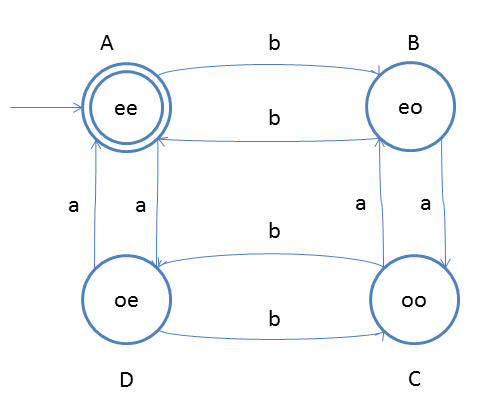# DFA String Examples

Design a DFA such a way that
Let string is w, Number of a(w) mod 2 = 0 and Number of b(w) mod 2 = 0. This means number of a should be divisible by 2 as well as number of b should also be divisible by 2.

As we approach to this question we have to first figure out the language which follows the above condition, and this is very easy task:
L = {Σ, aa, bb, bbaa, aabb, baba, ….}
Note This language is infinite

Think of the DFA we have designed earlier for number of a(w) mod 2 = 0 and number of b(w) mod 2 =0 , now we have to just combine the result.In the above pic you see one notation such ee, eo, oe and oo: they are for (even number of a, even number of b), (even number of a, odd number of b), (odd number of a, even number of b) and (odd number of a, odd number of b). So now if we traverse through the DFA using one string following the condition then we should end up on final stage. Note The notation ee, oe etc will make sense after a while, do not worry.

### Testing

1. Take string ababbb and traverse from left to right, here # a = 2 and #b = 4
2. from left first input is ‘a’ and start state ‘A’
3. so from state A we will go to state D
4. second input is ‘b’ and from D on b we will go to C
5. third input is ‘a’ and from C on a we will go state B
6. fourth input is ‘b’ and from B on b we will go to state A
7. fifth input is ‘b’ and from A on b we will go to state B
8. sixth input is ‘b’ and from B on b we will go to state A
After end of the string we are standing at state A, which is final state. So we say that string got accepted.